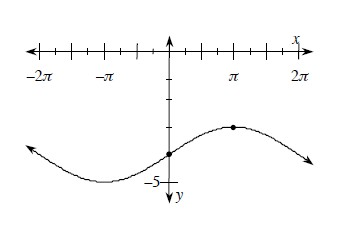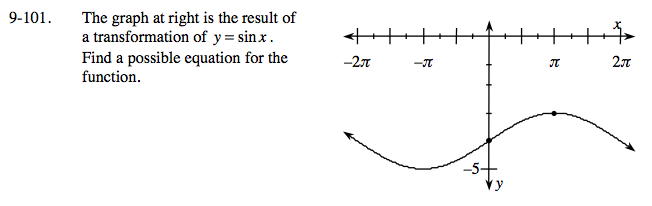### Home > PC > Chapter 9 > Lesson 9.3.1 > Problem9-101

9-101.
1. The graph below is the result of a transformation of y = sin x. Find a possible equation for the function. Homework Help ✎The vertical shift is down 4. There is no horizontal shift.

So a whole cycle (period) is 4π. Calculate the frequency b: bp = 2π, so 4πb = 2πb = ?

Substitute pertinent information into: y = Asin(b(x)) ± C